# Earnings per share (EPS)### Written byTrue Tamplin, BSc, CEPF®

Updated on December 21, 2022

## Earnings Per Share (EPS): Definition

Earnings per share (EPS) is a company’s net income divided by the average number of common shares outstanding during the year. It is also known as net income per share.

When a company allocates a portion of its profit to each common stock share, it is called earnings per share (EPS).

## EPS: Explanation

Earnings per share (EPS) is one of the most popular—if not the most popular—financial statistics reported in financial publications.

The relationship between EPS and stock market price is called the price-earnings ratio (P/E ratio or earnings multiple). It is usually shown alongside most stocks listed on the stock page in newspapers.

An understanding of how EPS is calculated, as well as its uses and limitations, is essential in order to become an informed user of financial information.

## Importance of Calculating EPS

As a way of summarizing performance for a particular time period, the generally accepted accounting principles (GAAP) require companies to report information regarding earnings per share (EPS).

Because this information is a highly condensed description of a large number of transactions and other events, it tends to be imperfect in communicating information to users.

Nonetheless, EPS is used extensively by participants in the capital markets, primarily to compare one company’s current results with those it achieved in the past and those achieved by other firms.

The ratio of stock price to EPS (known as the price-earnings ratio or earnings multiple) is used widely and often reported in daily listings of stock activity and prices. Additionally, the amount of dividends paid per share is often compared with EPS.

## The Basics: Calculating Earnings Per Share (EPS)

In the simplest sense, EPS is calculated by dividing net income available to common shareholders by the weighted average number of outstanding common shares.

### EPS Formula

The formula for EPS is shown as follows: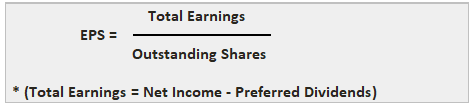EPS is meaningful only in regard to common stockholders. This is because preferred shareholders only have claims to the stipulated dividend.

### Net Income Available to Common Shareholders

Net income available to common shareholders is equal to net income less preferred stock dividends declared. If there is no preferred stock, the numerator of the formula will be simply net income.

To illustrate, assume that Mori Corporation earned a net income of \$1,562,500 in the year ended 31 December 2019. The firm declared the following dividends during 2019:

• Common dividends = \$125,000
• Preferred dividends = \$62,500

In this case, net income available to common shareholders is \$1,500,000, or \$1,562,500 - \$62,500.

The common dividends declared are ignored because all the earnings after preferred dividends are theoretically available to common shareholders whether or not they are returned in the form of dividends.

### Weighted Average Number of Common Shares Outstanding

If a corporation has not issued any additional common stock, the denominator of the earnings per share (EPS) formula will simply be the number of common shares outstanding at the end of the year.

In many cases, however, firms issue additional shares of common stock at various times during the year.

In these cases, it is incorrect to take the total number of shares outstanding at the end of the year because the firm did not benefit from the cash or other assets generated from the additional stock issue for the entire year.

Therefore, a weighted average number of shares must be calculated based on the number of months that the shares were outstanding.

To demonstrate, assume that Mori Corporation has 1 million shares of common stock outstanding on 1 January 2019. During the year, the firm issued the following additional common stock:The weighted average number of common shares outstanding is 1,225,000, calculated as follows: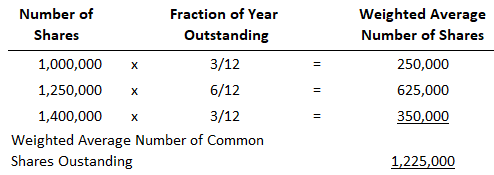In effect, the number of shares outstanding for any part of the year is multiplied by the fraction of the year in which they were outstanding. In a full year, 12/12ths must be accounted for.

Based on these data, EPS for Mori Corporation is \$1.22 (rounded), which is determined as follows: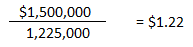An EPS figure of \$1.22 is more realistic than a figure determined using 1.4 million shares, the number of common shares outstanding at year-end.

Using 1.4 million shares would decrease the EPS. It would also be based on the assumption that the firm had used for the entire year of the cash from the additional stock issued on 1 April and 30 September.

### Calculating Earnings Per Share (EPS) for Complex Capital Structures

Many large corporations have what is referred to as complex capital structures. A complex capital structure contains certain types of bonds, preferred stock, or other securities that are convertible into common shares.

These types of convertible securities are often dilutive because if they were converted into common stock, thereby increasing the number of common shares outstanding, EPS could be reduced.

Accounting rules require that when a firm has a complex capital structure, two separate EPS figures must be calculated: primary EPS and fully diluted EPS.

### Accounting Practice

The overall goal of accounting practice concerning earnings per share (EPS) is to measure the increase in equity claims per common share that arose from operating events and other income-related activities in the given time period.

While this task might appear simple, it can be complex because of two major factors.

First, many types of securities and other contractual agreements have potentially significant effects on the number of shares of common stock. Examples are stock warrants, convertible bonds, and convertible preferred stock.

Second, creating uniformity among all firms leads to a far-reaching specification of assumptions that all firms are required to make, even if they do not really describe each individual firm’s situation.

Consequently, the EPS information presented on the income statement may be pro forma, or as if certain events occurred that really did not take place.

For example, it may be necessary to assume that convertible debt was converted even though—in reality—it wasn’t.

## Example 1

Suppose a company’s net income is \$15,000,000 and \$3,000,000 is paid to preferred stockholders. The company issues 19,000,000 outstanding shares. Calculate the earnings per share.

### Solution

First, we need to calculate the total earnings of common shareholders.

Total earnings = Net income - Preferred dividends Total earnings = \$15,000,000 - \$3,000,000 = \$12,000,000

Now, the earnings per share (EPS) figure is:

EPS = Total earnings / Outstanding shares EPS = \$12,000,000 / 19,000,000 = \$0.63

## Example 2

To illustrate the calculation of both primary and fully diluted EPS, suppose that GAAP Corporation, which earned a net income of \$2.5 million in 2019, has the capital structure shown in Exhibit A.

There are two separate issues of convertible preferred stock. Both are potentially dilutive, but we are assuming that only the 8% preferred stock is considered a common stock equivalent under the current accounting pronouncements.

The 6% preferred stock is not a common stock equivalent.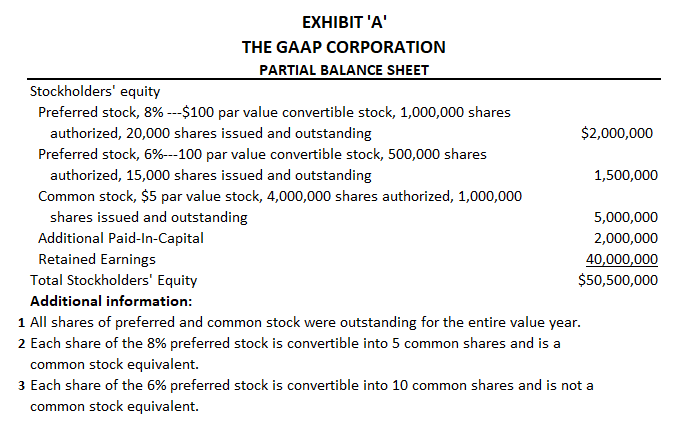Exhibit B shows how primary and fully diluted EPS are calculated.

In calculating primary EPS, net income available to common shareholders is determined by subtracting only the dividends on the 6% preferred stock—which is not a common stock equivalent—from the reported net income.

The 8% preferred stock—which is a common stock equivalent—is assumed to be converted into shares of common stock, and thus these dividends need not be subtracted from net income.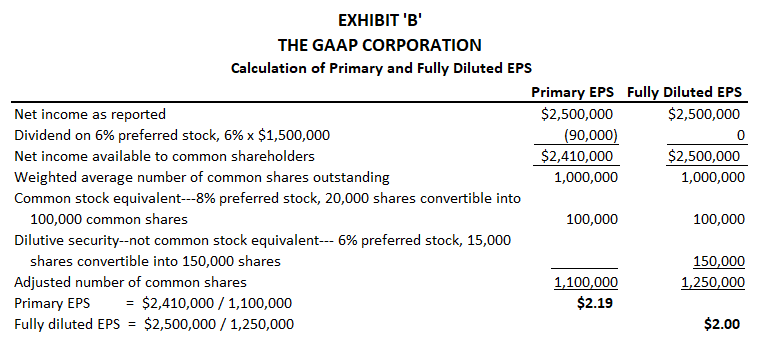As a result, the net income available to common shareholders is \$2,410,000.

The weighted average number of common shares at year-end is determined by adding the 1 million outstanding in common shares to the number of common shares that would be issued if the 8% preferred shares were converted.

In this case, we assume that the conversion happens at the beginning of the year.

Because each of the 20,000 preferred shares is convertible into 5 common shares, an additional 100,000 shares would be issued in this example. Thus, the adjusted number of common shares is 1.1 million and the primary EPS is \$2.19.

In calculating fully diluted EPS, we assume that all the convertible preferred shares, whether or not they are common stock equivalents, are converted into common shares.

Therefore, no adjustment is made to reported net income because now we assume that no preferred dividends will be paid. This is due to the fact that all the preferred stock is assumed to have been converted.

The weighted average number of common shares is determined by adding the 1 million outstanding shares of common to the number of shares that would be issued if both the 8% and 6% preferred shares were converted at the beginning of the year.

The adjusted number of common shares is 1.25 million and fully diluted EPS is \$2.

## Presenting Earnings Per Share (EPS) on Corporate Income Statements

All publicly held corporations are required to disclose EPS data on their income statements. Primary EPS must be disclosed, and if fully diluted EPS is at least 3% less than primary EPS, fully diluted EPS must also be disclosed.

EPS data should also be presented for major categories on the income statement, including income from continuing operations and discontinued operations.

Exhibit C shows an income statement from Kroger Company, a large food and drug retailer, including the relevant EPS disclosures.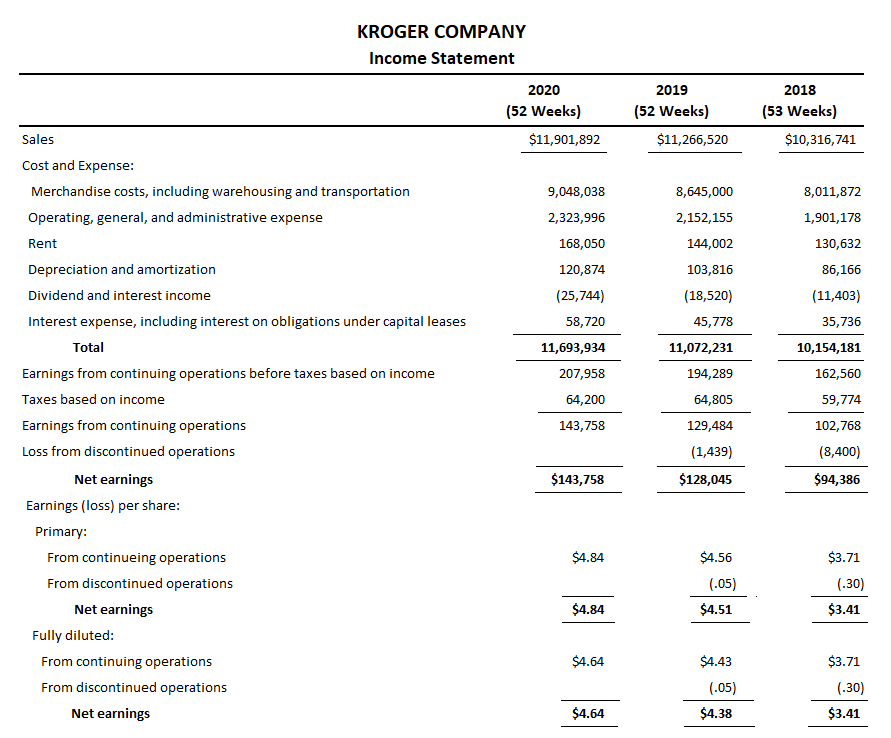## Uses and Limitations of Earnings Per Share (EPS) Data

Earnings per share (EPS) is a popular financial statistic that provides useful comparisons across companies. lt also provides a basis for other ratios such as the price-earnings ratio.

However, one must be extremely careful in using this data. As we noted, the rules for calculating EPS are complex, and it is often impossible for even a sophisticated user to calculate EPS from just looking at published financial data.

Furthermore, if you are relying on published EPS data, you still need to be careful.

For example, are the published price-earnings (P/E) ratios based on primary or fully diluted EPS? Is EPS from continuing operations used, or is the figure based on other income statement categories?

Generally, the P/E ratio is based on primary EPS calculated on income from continuing operations. These are only a few examples of the issues that must be considered when using EPS data.

### Relationship Between EBIT and EPS

Given that EPS is an important measure of a company’s performance, and since it is closely monitored by investors, finance managers pay special importance to it when making decisions.

It is the risk or the uncertainty factor involved in any decision that makes managers cautious. In fact, it is the responsibility of managers to enhance the benefits to the shareholders.

Since both EBIT and EPS are uncertain, managers cannot decide at once on a plan with the highest EPS. The reason is that a particular plan may provide greater EPS at one level of EBIT but less at another level.

This means that different financing decisions will have a varying impact on EPS. It is possible to examine the effects of various financing alternatives through EBIT-EPS analysis.

Let’s consider this relationship using an example.

#### Example

A company needs \$800,000 for its proposed new market entrance. It is evaluating the following alternative financial plans: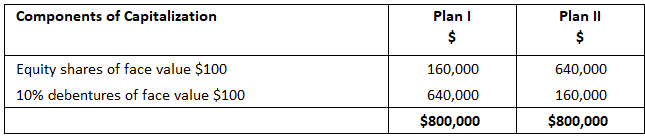With a tax rate of 30% applicable to the company, calculate the impact of EBIT and EPS when the earnings before interest and tax are \$80,000, \$120,000, and \$200,000.

#### Solution

1. When WBIT is \$80,000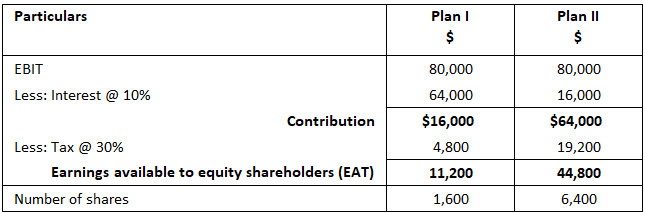EPS = Profit to equity holders / Number of equity shares = 11,200 / 1,600 = 7 = 44,800 / 6,400 = 7

2. When EBIT is \$120,000EPS = Profit to equity holders / Number of equity shares = 39,200 / 1,600 = 24.5 = 72,800 / 6,400 = 11.3

3. When EBIT is \$200,000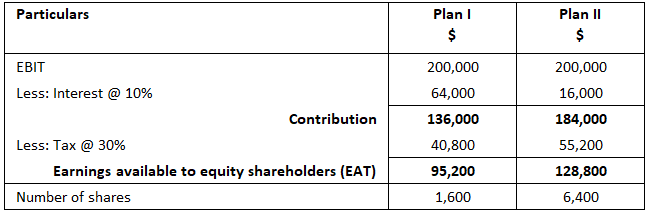EPS = Profit to equity holders /  Number of equity shares = 95,200 / 1,600 = 59.5 = 128,800 / 6,400 = 20.13

Inference: Although EPS remains the same at the \$80,000 EBIT level, you may notice that EPS increases when EBIT increases, but the rate of EPS differs with an EBIT of 120,000 and 200,000 due to the capital structure differences.

Moreover, the influence of fixed cost is not shown in this case. In conclusion, EPS increases with an increase in EBIT provided that the existing capital structure of the company remains unaltered.